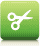PHP

# round()

## 实例

<?php
echo(round(0.60) . "<br>");
echo(round(0.50) . "<br>");
echo(round(0.49) . "<br>");
echo(round(-4.40) . "<br>");
echo(round(-4.60));
?>

## 定义和用法

round() 函数对浮点数进行四舍五入。

## 语法

round(number,precision,mode);

number 必需。规定要舍入的值。
precision 可选。规定小数点后的尾数。默认是 0。
mode 可选。规定表示舍入模式的常量：
• PHP_ROUND_HALF_UP - 默认。遇到 .5 的情况时向上舍入 numberprecision 小数位。舍入 1.5 到 2，舍入 -1.5 到 -2。
• PHP_ROUND_HALF_DOWN - 遇到 .5 的情况时向下舍入 numberprecision 小数位。舍入 1.5 到 1，舍入 -1.5 到 -1。
• PHP_ROUND_HALF_EVEN - 遇到 .5 的情况时取下一个偶数值舍入 numberprecision 小数位。
• PHP_ROUND_HALF_ODD - 遇到 .5 的情况时取下一个奇数值舍入 numberprecision 小数位。

## 技术细节

 返回值： 舍入后的值。 Float 4+ PHP 5.3：新增 mode 参数。## 实例 1

<?php
echo(round(4.96754,2) . "<br>");
echo(round(7.045,2) . "<br>");
echo(round(7.055,2));
?>

## 实例 2

<?php
echo(round(1.5,0,PHP_ROUND_HALF_UP) . "<br>");
echo(round(-1.5,0,PHP_ROUND_HALF_UP) . "<br>");

echo(round(1.5,0,PHP_ROUND_HALF_DOWN) . "<br>");
echo(round(-1.5,0,PHP_ROUND_HALF_DOWN) . "<br>");

echo(round(1.5,0,PHP_ROUND_HALF_EVEN) . "<br>");
echo(round(-1.5,0,PHP_ROUND_HALF_EVEN) . "<br>");

echo(round(1.5,0,PHP_ROUND_HALF_ODD) . "<br>");
echo(round(-1.5,0,PHP_ROUND_HALF_ODD));
?>

© 2014 bubuko.com 版权所有